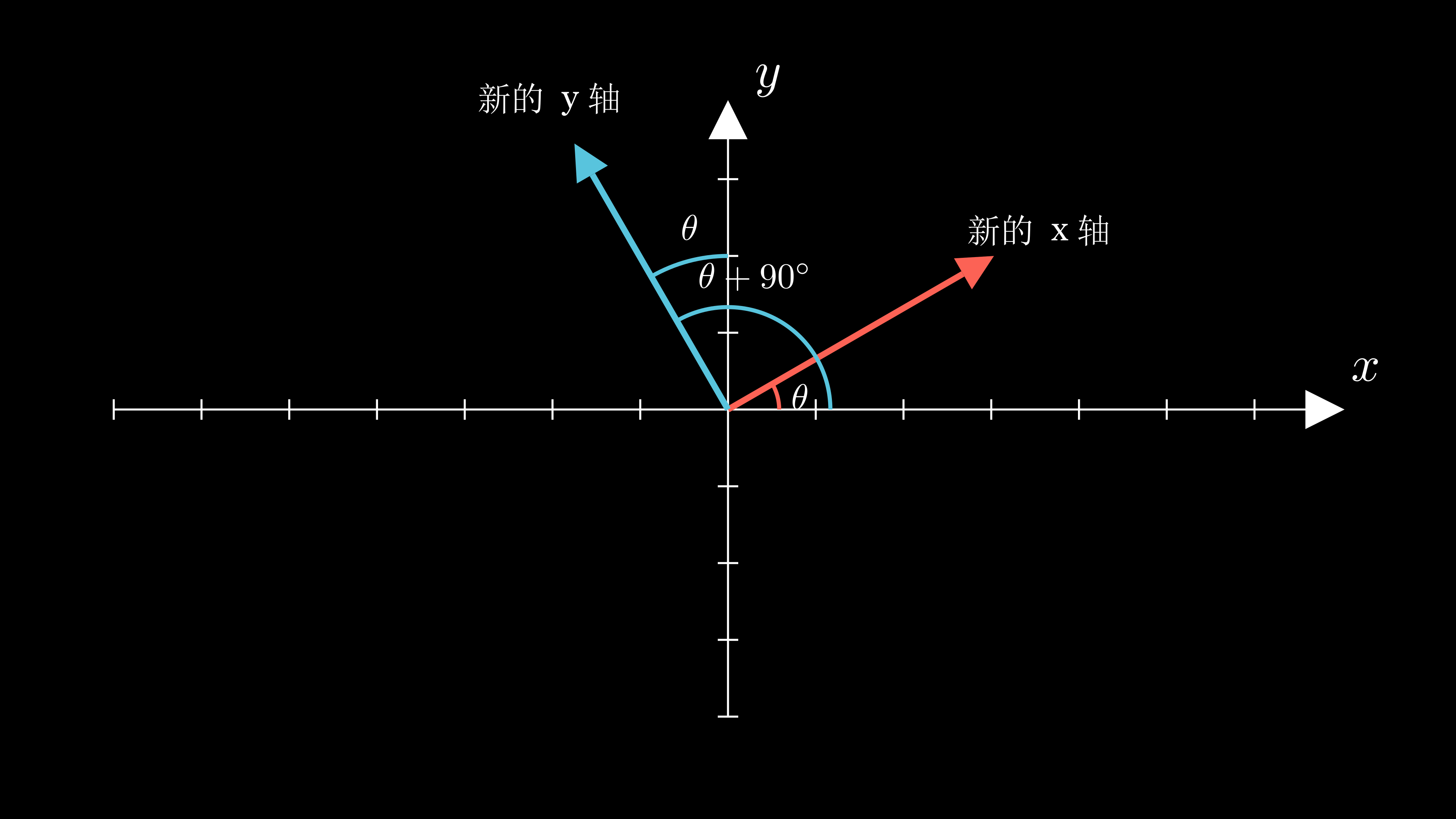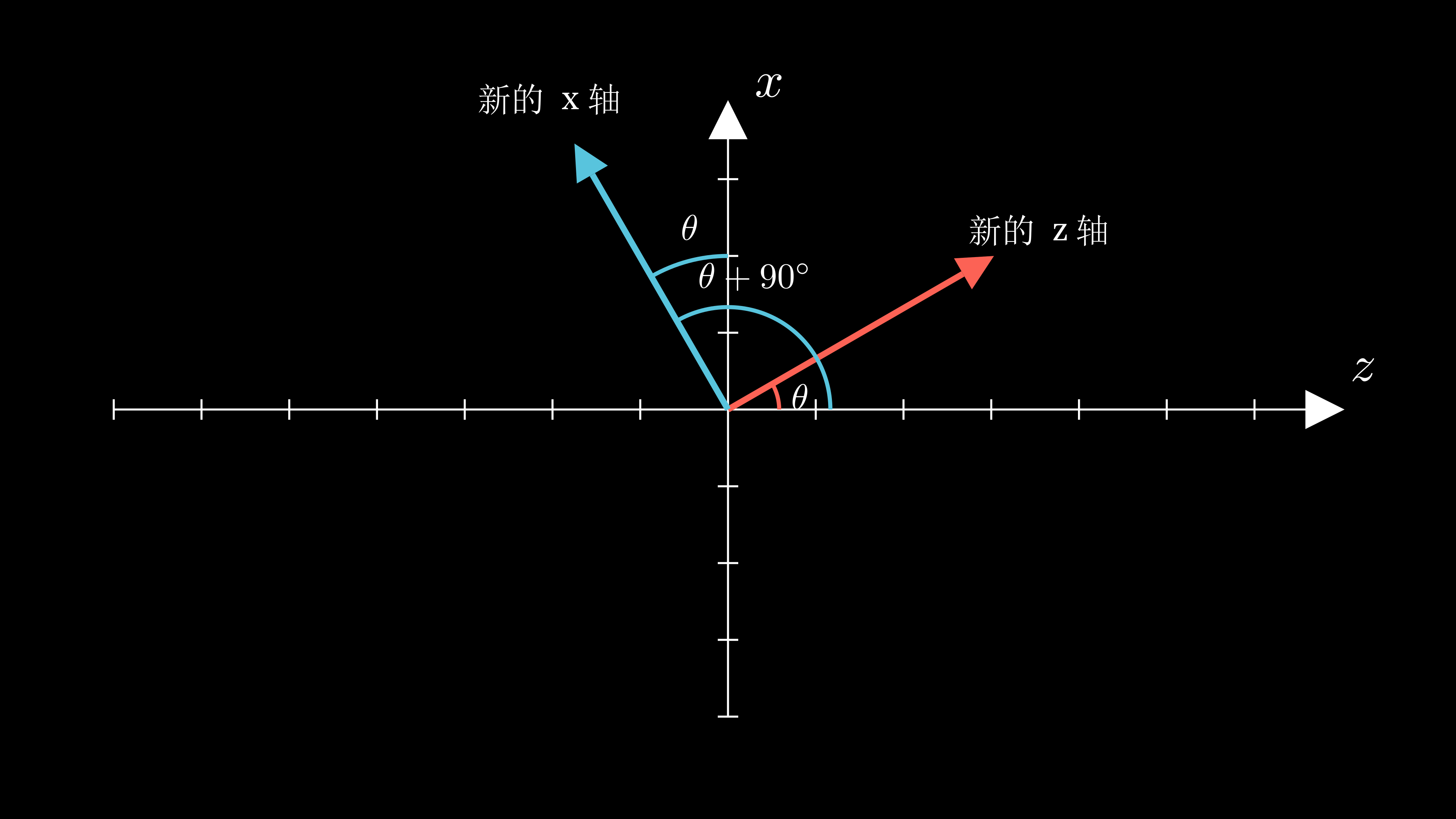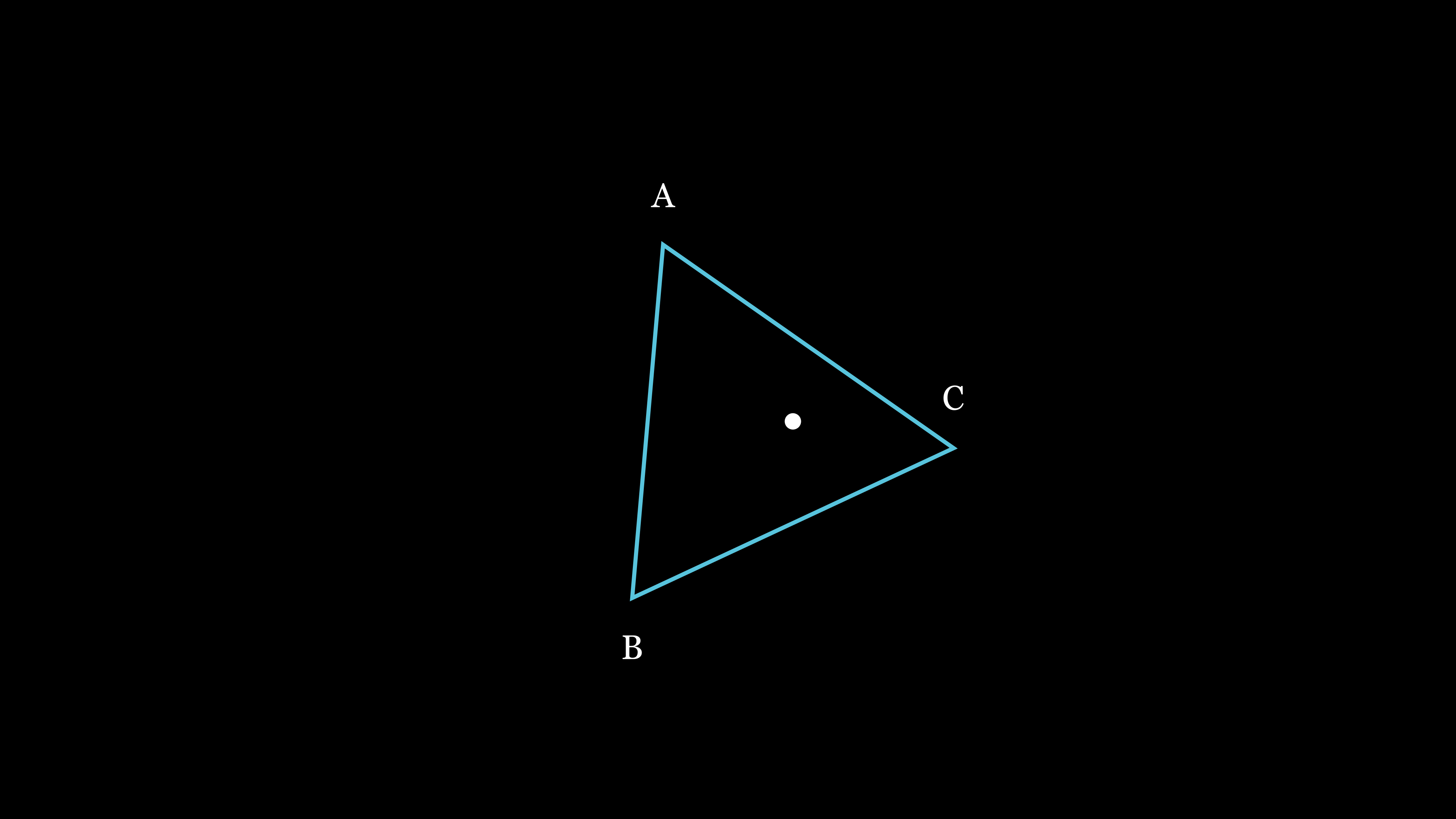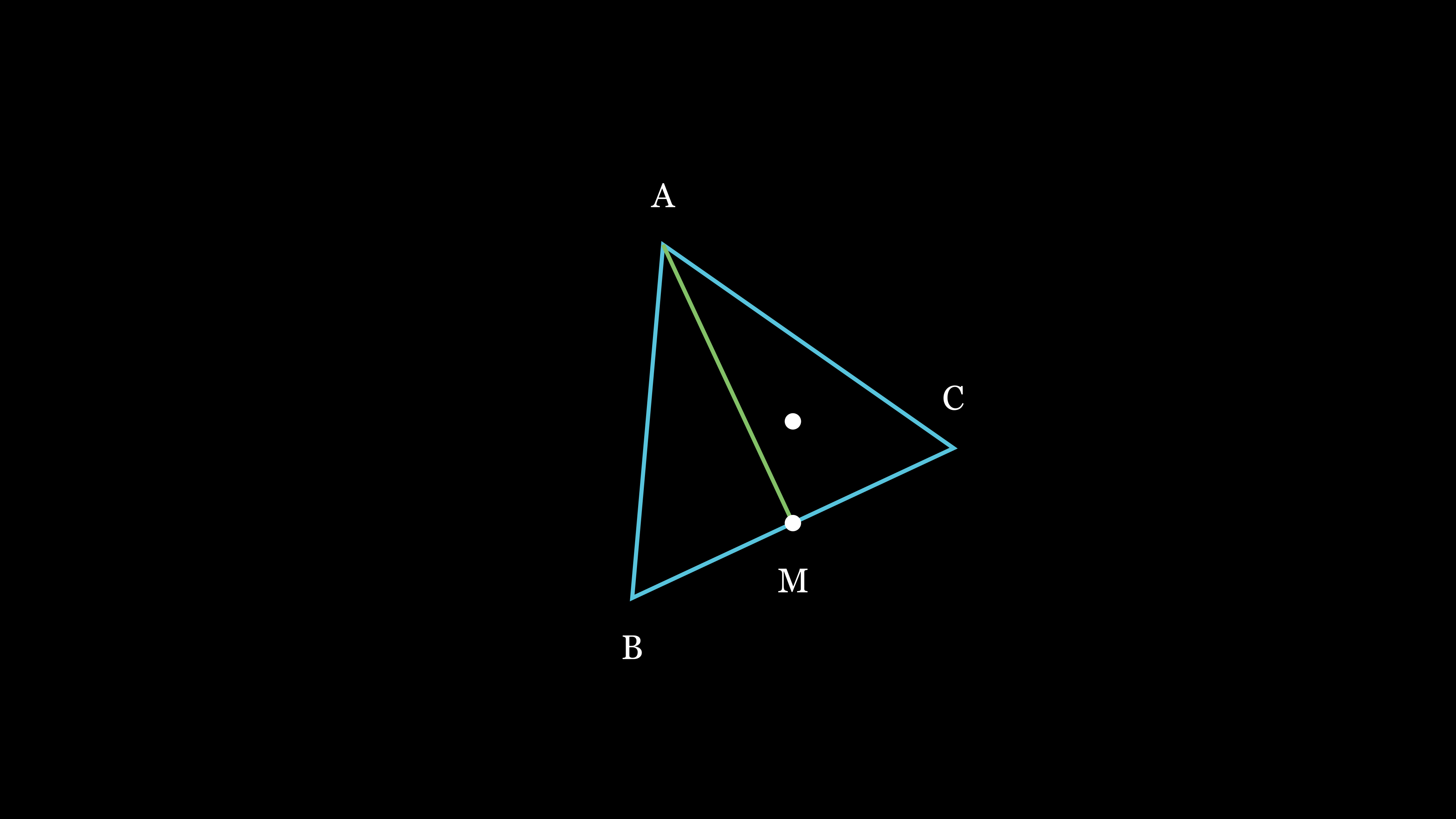# 三维旋矩阵

$R_x(\theta) = \begin{bmatrix} 1 & 0 & 0 \\ 0 & \cos \theta & -\sin \theta \\ 0 & \sin \theta & \cos \theta \end{bmatrix}$

$R_z(\theta) = \begin{bmatrix} \cos \theta & -\sin \theta & 0 \\ \sin \theta & \cos \theta & 0 \\ 0 & 0 & 1 \end{bmatrix}$

$R_y(\theta) = \begin{bmatrix} \cos \theta & 0 & \sin \theta \\ 0 & 1 & 0 \\ -\sin \theta & 0 & \cos \theta \end{bmatrix} \\$$\hat i = \begin{bmatrix} \cos \theta \\ \sin \theta \\ 0 \end{bmatrix} \\$

$\hat j = \begin{bmatrix} -\sin \theta \\ \cos \theta \\ 0 \end{bmatrix} \\$

$p = x\begin{bmatrix} \cos \theta \\ \sin \theta \\ 0 \end{bmatrix} + y\begin{bmatrix} -\sin \theta \\ \cos \theta \\ 0 \end{bmatrix} + z\begin{bmatrix} 0 \\ 0 \\ 1 \end{bmatrix}$

$\begin{bmatrix} \textcolor{red}{\cos \theta} & \textcolor{blue}{-\sin \theta} & \textcolor{green}{0} \\ \textcolor{red}{\sin \theta} & \textcolor{blue}{\cos \theta} & \textcolor{green}{0} \\ \textcolor{red}{0} & \textcolor{blue}{0} & \textcolor{green}{1} \end{bmatrix}\begin{bmatrix} x \\ y \\ z \\ \end{bmatrix} = x\textcolor{red}{\begin{bmatrix} \cos \theta \\ \sin \theta \\ 0 \end{bmatrix}} + y \textcolor{blue}{\begin{bmatrix} -\sin \theta \\ \cos \theta \\ 0 \end{bmatrix} } + z\textcolor{green}{\begin{bmatrix} 0 \\ 0 \\ 1 \end{bmatrix}}$$\hat k = \begin{bmatrix} \sin \theta \\ 0 \\ \cos \theta \end{bmatrix} \\$

$\hat i = \begin{bmatrix} \cos \theta \\ 0 \\ -\sin \theta \end{bmatrix} \\$

$\hat j = \begin{bmatrix} 0 \\ 1 \\ 0 \end{bmatrix} \\$

$\left[\hat i \ | \ \hat j \ | \ \hat k\right] = \begin{bmatrix} \cos \theta & 0 & \sin \theta \\ 0 & 1 & 0 \\ -\sin \theta & 0 & \cos \theta \end{bmatrix} \\$

# 重心坐标

GAMES101 课程中讲的一样，重心坐标（特别是三角形的）在图形学中非常有用，可以很方便的把三角形节点上的信息插值到三角形面上。

## 物理角度

$\text{重心} = \frac{M_aA + M_bB + M_cC}{M_a + M_b + M_c}$

$A\frac{M_a}{M_a + M_b + M_c} + B\frac{M_b}{M_a + M_b + M_c} + C\frac{M_c}{M_a + M_b + M_c}$

## 代数角度$p = A + u\overrightarrow{AB} + v\overrightarrow{AC}$

$p - A = u\overrightarrow{AB} + v\overrightarrow{AC} \\ \overrightarrow{pA} = u\overrightarrow{AB} + v\overrightarrow{AC} \\ u\overrightarrow{AB} + v\overrightarrow{AC} - \overrightarrow{pA} = {0}$

\begin{align*} u(B - A) + v(C - A) + A - p &= 0 \\ uB - uA + vC - vA + A - p &= 0\\ A(1 - u - v) + uB + vC - p &= 0 \\ (1 - u - v)A + uB + vC &= p \end{align*}$\overrightarrow{AB}$$\overrightarrow{AM}$ 上的投影长度是 $u|\overrightarrow{AM}|$$\overrightarrow{AC}$$\overrightarrow{AM}$ 上的投影长度是 $v|\overrightarrow{AM}|$，自然，这两个向量的和在 $\overrightarrow{AM}$ 上的投影长度就是 $(u + v)|\overrightarrow{AM}|$